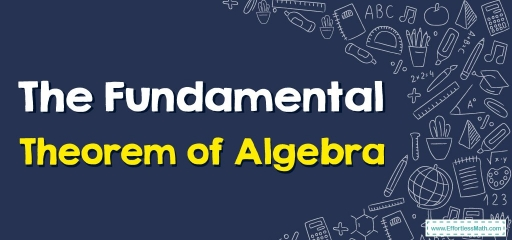# The Fundamental Theorem of Algebra

The Fundamental Theorem of Algebra states that every polynomial equation of degree $$n$$ with complex number coefficients has $$n$$ roots, or solutions, in the complex numbers.The Fundamental Theorem of Algebra is an example of an ‘existence’ theorem in mathematics, like the Intermediate Value Theorem. This theorem guarantees the existence of at least one zero but gives us no algorithm to find it.

## A step-by-step guide to the fundamental theorem of algebra

Every polynomial function contains at least one complex zero, according to the Fundamental Theorem of Algebra. The cornerstone for solving polynomial equations is this theorem.

There is at least one complex root in every polynomial equation with complex coefficients and degrees. Gauss was the first to prove this theorem. It’s the same as saying that a polynomial $$P(z)$$ of degree $$n$$ has $$n$$ values $$z_i$$ (some of them possibly degenerate) for which $$P(z_i)=0$$. Polynomial roots are the names given to such numbers.

An example of a polynomial with a single root of multiplicity $$>1$$ is $$z^2-2z+1=(z-1)(z-1)$$ which has $$z=1$$ as a root of multiplicity $$2$$.

### The Fundamental Theorem of Algebra – Example 1:

what are the roots of $$x^2-4$$?

$$x^2-4$$ has a degree of $$2$$ (the largest exponent of $$x$$ is $$2$$), so there are 2 roots.

Let us solve it. We want it to be equal to zero:

$$x^2-4=0$$

$$x^2=4$$

Now, take the square root of both sides:

$$x=\pm2$$

So, the roots are $$+2$$ and $$-2$$.

## Exercises for the Fundamental Theorem of Algebra

1. Write the polynomial that has the following roots $$4$$ (with multiplicity $$3$$), $$2$$ (with multiplicity $$2$$) and $$0$$.
2. Find the zeroes of the polynomial $$x^2+3x+2$$.
3. Find the zeroes of the polynomial $$x^3-4x^2-4x+16$$.
4. How many roots does the following equation have? $$\left(2x^2+2x-1\right)\left(3x^2-9\right)=0$$
1. $$\color{blue}{f(x)=(x-4)^3(x-2)^2 x}$$
2. $$\color{blue}{x=-1, \:or\: x=-2}$$
3. $$\color{blue}{x=2, x=-2 \:or\: x=4}$$
4. $$\color{blue}{4}$$

### What people say about "The Fundamental Theorem of Algebra - Effortless Math: We Help Students Learn to LOVE Mathematics"?

No one replied yet.

X
30% OFF

Limited time only!

Save Over 30%

SAVE $5 It was$16.99 now it is \$11.99